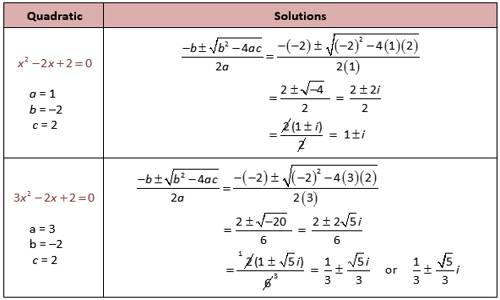A polynomial of the second degree is generally called a quadratic polynomial. In elementary algebra, the quadratic formula is the solution of the quadratic equation. There are other ways to solve the quadratic equation instead of using the quadratic formula, such as factoring, completing the square, or graphing. Using the quadratic formula is often the most convenient way.

If f(x) is a quadratic polynomial, then f(x) = 0 is called a quadratic equation.

An equation in one unknown quantity in the form ax2 + bx + c = 0 is called quadratic equation.

A quadratic equation is an equation of the second degree.

Complex Roots of a Quadratic Equation

In a quadratic equation with real coefficients has a complex root α + iβ then it has also the conjugate complex root α – iβ.

Proof:

To prove the above theorem let us consider the quadratic equation of the general form:

ax2 + bx + c = 0 where, the coefficients a, b and c are real.

Let α + iβ (α, β are real and i = √-1) be a complex root of equation ax2 + bx + c = 0. Then the equation ax2 + bx + c = 0 must be satisfied by x = α + iβ.

Therefore,

a(α + iβ)2 + b(α + iβ) + c = 0

or, a(α2 – β2 + i ∙ 2 αβ) + bα + ibβ + c = 0, (Since, i2 = -1)

or, aα2 – aβ2 + 2iaαβ + bα + ibβ + c = 0,

or, aα2 – aβ2 + bα + c + i(2aαβ + bβ) = 0,

Therefore,

2 – aβ2 + bα + c = 0 and 2aαβ + bβ = 0

Since, p + iq = 0 (p, q are real and i = √-1) implies p = 0 and q = 0]

Now substitute x by α – iβ in ax2 + bx + c we get,

a(α – iβ)2 + b(α – iβ) + c

= a(α2 – β2 – i ∙ 2 αβ) + bα – ibβ + c, (Since, i2 = -1)

= aα2 – aβ2 – 2iaαβ + bα – ibβ + c,

= aα2 – aβ2 + bα + c – i(2aαβ + bβ)

= 0 – i ∙ 0 [Since, aα2 – aβ2 + bα + c = 0 and 2aαβ + bβ = 0]

= 0

Now we clearly see that the equation ax2 + bx + c = 0 is satisfied by x = (α – iβ) when (α + iβ) is a root of the equation. Therefore, (α – iβ) is the other complex root of the equation ax2 + bx + c = 0.

Similarly, if (α – iβ) is a complex root of equation ax2 + bx + c = 0 then we can easily proved that its other complex root is (α + iβ).

Thus, (α + iβ) and (α – iβ) are conjugate complex roots. Therefore, in a quadratic equation complex or imaginary roots occur in conjugate pairs.Solved example to find the imaginary roots occur in conjugate pairs of a quadratic equation:

Find the quadratic equation with real coefficients which has 3 – 2i as a root (i = √-1).

Solution:

According to the problem, coefficients of the required quadratic equation are real and its one root is 3 – 2i. Hence, the other root of the required equation is 3 – 2i (Since, the complex roots always occur in pairs, so another root is 3 + 2i.

Now, the sum of the roots of the required equation = 3 – 2i + 3 + 2i = 6

And, product of the roots = (3 + 2i)(3 – 2i) = 32 – (2i)2 = 9 – 4i2 = 9 -4(-1) = 9 + 4 = 13

Hence, the equation is

x2 – (Sum of the roots)x + product of the roots = 0

i.e., x2 – 6x + 13 = 0

Therefore, the required equation is x2 – 6x + 13 = 0.

Information Source: